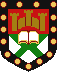# Classical Meaning of Potential

Put simply, the potential is just the energy per unit charge which a test particle of negligible charge will possess due to its position in a particular field. Two points should be noted concerning that last statement. First, I am using the word charge in its widest sense; for example, if one wants to describe a falling ball, then the field in question is the gravitational field and the corresponding charge is the ball's mass. In an electric field the charge would be the familiar electromagnetic charge. Secondly, I will restrict this discussion to conservative fields where the work done in moving the test charge from one particular position to another is independant of the path taken and of the velocity of the test charge.

Let us consider a simple example. Since it requires energy to lift an object through a gravitational field it follows that the potential of an object in the Earth's gravitational field must increase with height. In fact, the gravitational potential close to the Earth's surface is directly proportional to the height above any arbitrary reference position.

Now, it is a fundamental law of nature that systems always tend towards their lowest potential energy configuration, within the constraint of conservation of energy. This means that an object in any given field will always feel a force in the direction of maximum decrease of the potential. The magnitude of this force will be directly proportional to the rate of decrease of potential in this direction. Thus a ball close to the surface of the Earth will always experience a constant gravitational force directly downwards (downwards because this is the direction of maximum potential decrease, and constant because the rate of decrease is constant). Electrical and other fields work in just the same way, except they act on charges other than mass. That is, in order to describe the motion of a classical particle it is only necessary to know the potential in which it moves and its charge. Forces can be simply worked out from the rate of change of the potential with position.

The potential is convenient for describing the environment of a quantum mechanical particle because it allows us to assign a simple scalar quantity to each point in space, rather than keeping track of vector forces acting on a particle whose position may not be precisely known.

Stephen Jenkins